# Top 2-way interaction strength for Fit Model and Discover Key Predictors with TreeNet® Regression

###### Note

Assume that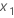and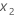are two predictor variables. Minitab provides two measures of the strengths of interactions. The measures come from the training data. The first measure describes the strength of the interaction as a percentage of the total response squared deviation:
The second measure describes the strength of the interaction as a percentage of the response squared deviation from the variables in the interaction:
From the training data, the total squared deviation of the response surface is as follows:

where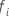is the fitted value from the TreeNet model and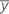is the mean of the response variable.

The calculation for the deviation of the interaction requires the calculation of the deviation for both variables jointly. The joint calculation for the deviations has the following form:

where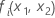is the fitted value from a bivariate partial dependence surface. For the calculation of this fitted value, go to Partial dependence plots for Fit Model and Discover Key Predictors with TreeNet® Regression.

The calculation for the deviation of the interaction removes the main effects from the joint calculation:

where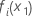and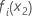are fitted values from univariate partial dependence surfaces forand. For the calculation of these fitted values, go to Partial dependence plots for Fit Model and Discover Key Predictors with TreeNet® Regression.